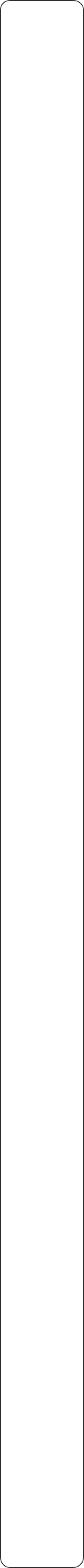Year 2 Objectives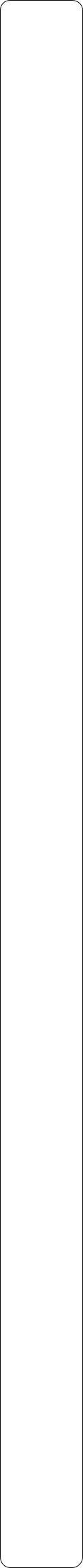Place ValuePre Tasks

(PitStops)

Main Tasks

Addition and Subtraction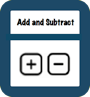Pre Tasks

(PitStops)

Main TasksMultiplication and Division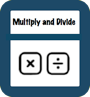Number - Fractions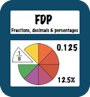Pre Tasks

(PitStops)

Main Tasks

Pre Tasks

(PitStops)

Main Tasks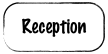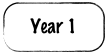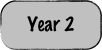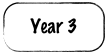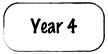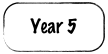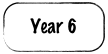- Count in steps of 2, 3, and 5 from 0, and in tens from any number, forwards and backwards

- Recognise the place value of each digit in a two-digit number (tens, ones)

1. -Identify, represent and estimate numbers using different representations, including the number line

1. -Compare and order numbers from 0 up to 100; use <, > and = signs

1. -Read and write numbers to at least 100 in numerals and in words

Use place value and number facts to solve problems

- Using concrete objects and pictorial representations, including those involving numbers, quantities and measures

solve problems with addition and subtraction:

- Applying their increasing knowledge of mental and written methods

- Recall and use addition and subtraction facts to 20 fluently, and derive and use related facts up to 100

Add and subtract numbers using concrete objects, pictorial representations, and mentally, including:

- A two-digit number and ones

- A two-digit number and tens

- Two two-digit numbers

- Adding three one-digit numbers

- Show that addition of two numbers can be done in any order (commutative) and subtraction of one number from another cannot

- Recognise and use the inverse relationship between addition and subtraction and use this to check calculations and solve missing number problems.

- Recall and use multiplication and division facts for the 2, 5 and 10 multiplication tables, including recognising odd and even number

- Calculate mathematical statements for multiplication and division within the multiplication tables and write them using the multiplication (×), division (÷) and equals (=) signs

- Show that multiplication of two numbers can be done in any order (commutative) and division of one number by another cannot

- Solve problems involving multiplication and division, using materials, arrays, repeated addition, mental methods, and multiplication and division facts, including problems in contexts

1. -Recognise, find, name and write fractions ⅓, ¼, ¾ of a length, shape, set of objects or quantity

Pupils should be taught to:

- Write simple fractions for example  ½ of 6 = 3 and recognise the equivalence of 2/4 and ½

Solutions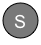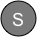StopsGap

StopsGap

StopsGap

StopsGap